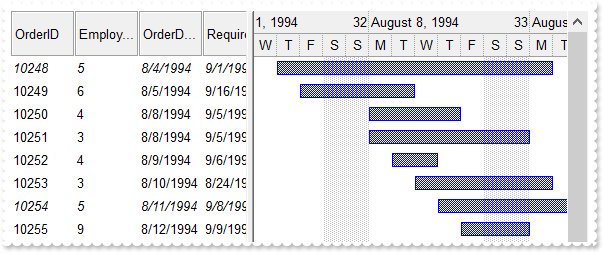property ConditionalFormat.Italic as Boolean
Specifies whether the objects that match the condition should appear in italic.Type Description Boolean A boolean expression that indicates whether the objects should look in italic.
The ApplyTo property specifies whether the ConditionalFormat object is applied to items or to cells in the column.

The following screen shot shows the control when the Italic property is set:The following VB sample makes italic the cells in the second column ( 1 ), if the sum between second and third column ( 2 ) is less than the value in the first column ( 0 ):

```With G2antt1.ConditionalFormats.Add("%1+%2<%0")
.ApplyTo = 1
.Italic = True
End With```

The following C++ sample makes italic the cells in the second column ( 1 ), if the sum between second and third column ( 2 ) is less than the value in the first column ( 0 ):

```COleVariant vtEmpty;
CConditionalFormat cf = m_g2antt.GetConditionalFormats().Add( "%1+%2<%0", vtEmpty );
cf.SetItalic( TRUE );
cf.SetApplyTo( 1 );```

The following VB.NET sample makes italic the cells in the second column ( 1 ), if the sum between second and third column ( 2 ) is less than the value in the first column ( 0 ):

```With AxG2antt1.ConditionalFormats.Add("%1+%2<%0")
.ApplyTo = 1
.Italic = True
End With```

The following C# sample makes italic the cells in the second column ( 1 ), if the sum between second and third column ( 2 ) is less than the value in the first column ( 0 ):

```EXG2ANTTLib.ConditionalFormat cf = axG2antt1.ConditionalFormats.Add("%1+%2<%0",null);
cf.Italic = true;
cf.ApplyTo = (EXG2ANTTLib.FormatApplyToEnum)1;```

The following VFP sample makes italic the cells in the second column ( 1 ), if the sum between second and third column ( 2 ) is less than the value in the first column ( 0 ):

```with thisform.G2antt1.ConditionalFormats.Add("%1+%2<%0")
.Italic = .t.
.ApplyTo = 1
endwith```